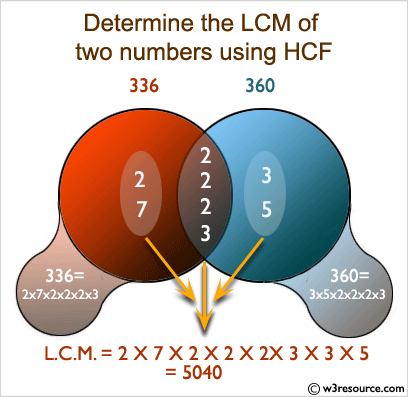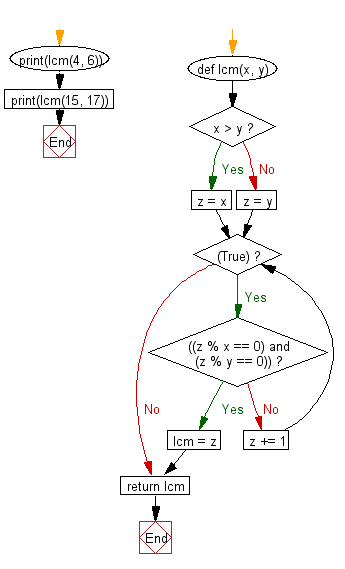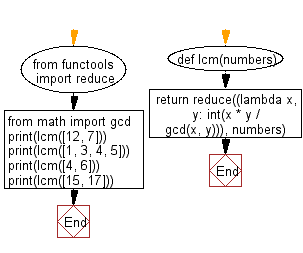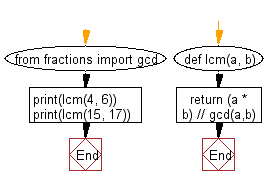﻿ Python: Get the least common multiple (LCM) of two positive integers - w3resource# Python: Get the least common multiple (LCM) of two positive integers

## Python Basic: Exercise-32 with Solution

Write a Python program to get the least common multiple (LCM) of two positive integers.

From Wikipedia,
In arithmetic and number theory, the least common multiple, lowest common multiple, or smallest common multiple of two integers a and b, usually denoted by lcm(a, b), is the smallest positive integer that is divisible by both a and b. Since division of integers by zero is undefined, this definition has meaning only if a and b are both different from zero. However, some authors define lcm(a,0) as 0 for all a, which is the result of taking the lcm to be the least upper bound in the lattice of divisibility.

Pictorial Presentation:Sample Solution-1:

Python Code:

``````def lcm(x, y):
if x > y:
z = x
else:
z = y
while(True):
if((z % x == 0) and (z % y == 0)):
lcm = z
break
z += 1
return lcm
print(lcm(4, 6))
print(lcm(15, 17))
```
```

Sample Output:

```12
255
```

Flowchart:## Visualize Python code execution:

The following tool visualize what the computer is doing step-by-step as it executes the said program:

Sample Solution-2:

Use functools.reduce(), math.gcd() and lcm(x,y) = x * y / gcd(x,y) over the given list.

Python Code:

``````from functools import reduce
from math import gcd

def lcm(numbers):
return reduce((lambda x, y: int(x * y / gcd(x, y))), numbers)

print(lcm([12, 7]))
print(lcm([1, 3, 4, 5]))
print(lcm([4, 6]))
print(lcm([15, 17]))
```
```

Sample Output:

```84
60
12
255
```

Flowchart:## Visualize Python code execution:

The following tool visualize what the computer is doing step-by-step as it executes the said program:

Sample Solution-3:

Python Code:

``````from fractions import gcd
def lcm(a, b):
return (a * b) // gcd(a,b)
print(lcm(4, 6))
print(lcm(15, 17))
```
```

Sample Output:

```12
255
```

Flowchart:## Visualize Python code execution:

The following tool visualize what the computer is doing step-by-step as it executes the said program:

Python Code Editor:

Have another way to solve this solution? Contribute your code (and comments) through Disqus.

What is the difficulty level of this exercise?

Test your Python skills with w3resource's quiz

﻿

## Python: Tips of the Day

List comprehension:

```>>> m = [x ** 2 for x in range(5)]
>>> m
[0, 1, 4, 9, 16]
```